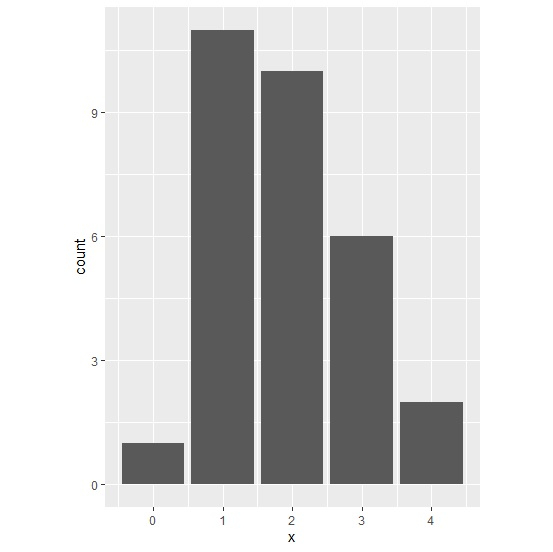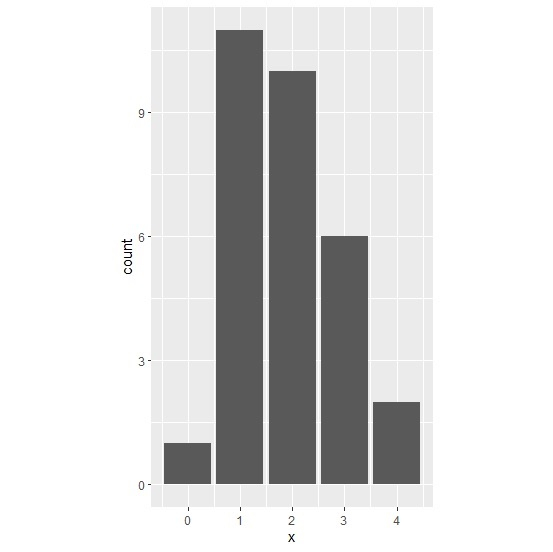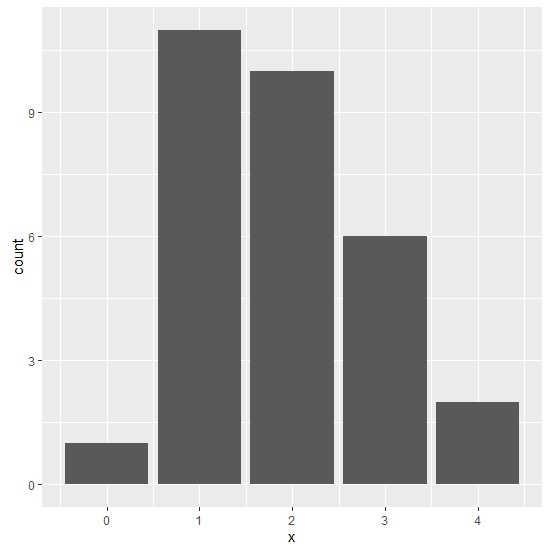# How to change the aspect ratio of a plot in ggplot2 in R?

The aspect ratio of a chart can be changed in ggplot2 and this will be useful if we want a smaller image of the chart. Sometimes, we don’t have large space where the chart will be pasted therefore this functionality becomes useful. Mostly, in research reports we see charts that are of small size, hence R becomes helpful to create charts that can be pasted in the desired space. This can be done with the help of theme function.

## Example

Consider the below data frame −

> set.seed(100)
> x<-rpois(30,2)
> df<-data.frame(x)

> library(ggplot2)

Creating the plot with aspect ratio 4/3 −

> ggplot(df,aes(x))+
+ geom_bar()+
+ theme(aspect.ratio=4/3)

## OutputCreating the plot with aspect ratio 16/9 −

> ggplot(df,aes(x))+
+ geom_bar()+
+ theme(aspect.ratio=16/9)

## OutputCreating the plot with aspect ratio 1, it gives us a square form −

> ggplot(df,aes(x))+
+ geom_bar()+
+ theme(aspect.ratio=1)

## Output